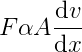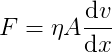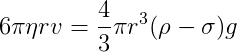Viscosity of a liquid - Stoke's method

# Objective

To determine the coefficient of viscosity of a given viscous liquid by measuring terminal velocity of a given spherical body.

# Theory

## How do you define viscosity?

Viscosity is the property of a fluid by virtue of which an internal resistance comes into play when the liquid is in motion, and opposes the relative motion between its different layers. Thus, it is the resistance of a fluid to flow.

When liquid flows over flat surface, a backward viscous force acts tangentially to every layer. This force depends upon the area of the layer, velocity of the layer, and the distance of the layer from the surface.Where η is the coefficient of viscosity of the liquid.

## Stoke’s Law

Stoke’s law was established by an English scientist Sir George G Stokes (1819-1903).

When a spherical body moves down through an infinite column of highly viscous liquid, it drags the layer of the liquid in contact with it. As a result, the body experiences a retarding force.

Then according to Stokes law, the viscous drag force,,

where,  r - Radius of the spherical body

v - Velocity of the spherical body

It gives the relationship between retarding force and velocity. When viscous force plus buoyant force becomes equal to force due to gravity, the net force becomes zero. The sphere then descends with a constant terminal velocity (v t).

Now,where,  ρ - Density of the liquid

σ - Density of the spherical body

# Learning Outcomes

• Students understand the behavior and properties of fluids
• Students get the knowledge about viscosity of liquids.
• Students understand the quantity, coefficient of viscosity and the various factors affecting its value.
• Students get the concept of terminal velocity.